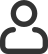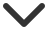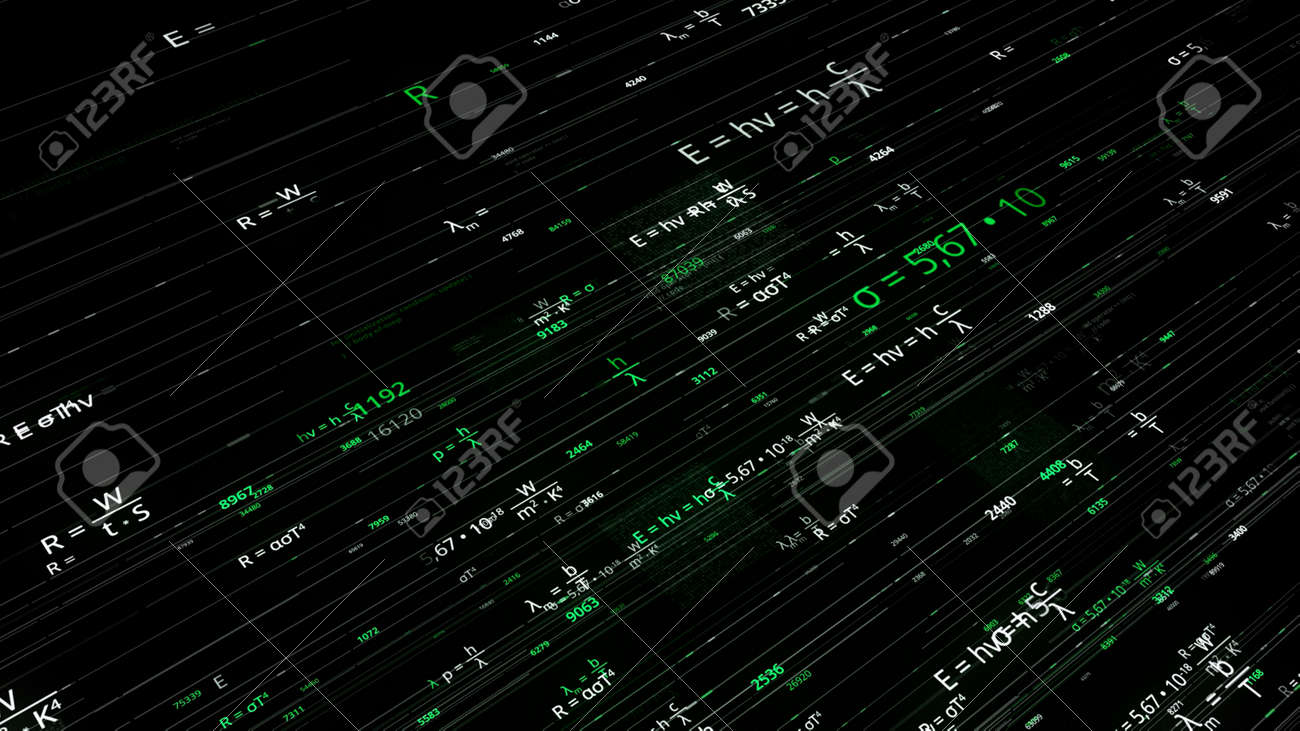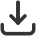Merhaba, Guest
Diller
Türkçe# Stok Fotoğraf — Mathematical formulas in matrix. Animation. Rewritable mathematical formulas in computer matrix networks. Scientific Foundation with Mathematical EquationsBoyut
Standart Boyutlar
S923 x 519 px • 72 dpi923 x 519 px12.82 x 7.21 inches
M2731 x 1536 px • 300 dpi2731 x 1536 px9.1 x 5.12 inches
L3840 x 2160 px • 300 dpi3840 x 2160 px12.8 x 7.2 inches
XL5760 x 3240 px • 300 dpi5760 x 3240 px19.2 x 10.8 inches
Görüntü bilgisi## Mathematical formulas in matrix. Animation. Rewritable mathematical formulas in computer matrix networks. Scientific Foundation with Mathematical Equations

Görsel Kimliği: 169163147
Medya Türü: Stok Fotoğraf
Telif hakkı: davidoff205020